# Water Quality Monitoring: Estimation of Alkalinity, BOD & COD Notes | Study Environmental Engineering - Civil Engineering (CE)

## Civil Engineering (CE): Water Quality Monitoring: Estimation of Alkalinity, BOD & COD Notes | Study Environmental Engineering - Civil Engineering (CE)

The document Water Quality Monitoring: Estimation of Alkalinity, BOD & COD Notes | Study Environmental Engineering - Civil Engineering (CE) is a part of the Civil Engineering (CE) Course Environmental Engineering.
All you need of Civil Engineering (CE) at this link: Civil Engineering (CE)

ALKALINITY (AT)

• Alkalinity is a measure of the ability of a solution to neutralize acids to the equivalence point of carbonate or bicarbonate. It is the water’s ability to absorb hydrogen ions without significant pH change. Alkalinity is a measure of the buffering capacity of water.
• Alkalinity is equal to the stoichiometric sum of the bases in solution.
• In natural environment, carbonate alkalinity makes up most of the total alkalinity due to the common occurrence and dissolution of carbonate rocks and presence of carbon dioxide in the atmosphere.
• Other natural components that contribute to alkalinity include hydroxide, borate, phosphate, silicate, nitrate, dissolved ammonia, conjugate bases of some organic acids and sulfide.
• Alkalinity is usually expressed in meq/L (milliequivalent per liter).

Alkalinity ( mol L)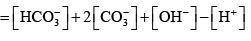(1.6.1)

Where the quantities in parenthesis are concentrations in meq/L or mg/L as CaCO3

( mg L) of X as CaCO3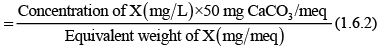BIOCHEMICAL OXYGEN DEMAND (BOD)

•  Biochemical Oxygen Demand (BOD) is a chemical procedure for determining how fast biological organisms use up oxygen in a body of water.
• It is used in water quality management and assessment, ecology and environmental science.
• BOD is not an accurate quantitative test, although it is considered as an indication of the quality of a water source.
• It is most commonly expressed in milligrams of oxygen consumed per litre of sample during 5 days of incubation at 20 °C or 3 days of incubation at 27 °C.
• The BOD test must be inhibited to prevent oxidation of ammonia. If the inhibitor is not added, the BOD will be between 10% and 40% higher than can be accounted for by carbonaceous oxidation .

Stages of Decomposition in the BOD test

• There are two stages of decomposition in the BOD test: a carbonaceous stage and a nitrogenous stage.
• The carbonaceous stage represents oxygen demand involved in the conversion of organic carbon to carbon dioxide.
• The second stage or the nitrogenous stage represents a combined carbonaceous plus nitrogenous demand, when organic nitrogen, ammonia and nitrite are converted to nitrate. Nitrogenous oxygen demand generally begins after about 6 days.
• Under some conditions, if ammonia, nitrite, and nitrifying bacteria are present, nitrification can occur in less than 5 days. In this case, a chemical compound that prevents nitrification is added to the sample if the intent is to measure only the carbonaceous demand. The results are reported as carbonaceous BOD (CBOD) or as CBOD5 when a nitrification inhibitor is used.

BOD – Dilution Method: BOD is the amount of oxygen (Dissolved Oxygen (DO)) required for the biological decomposition of organic matter. The oxygen consumed is related to the amount of biodegradable organics.
When organic substances are broken down in water, oxygen is consumed
Organic Carbon + O2 → CO2
Where, organic carbon in human waste includes protein, carbohydrates, fats, etc

Measure of BOD = Initial oxygen- Final Oxygen after (5 days at 20 °C) or (3 days at 27 °C)

Two standard 300 mL BOD bottles are filled completely with wastewater. The bottles are sealed. Oxygen content (DO) of one bottle is determined immediately. The other bottle is incubated at 20 oC for 5 days or (or at 27 °C for 3 days) in total darkness to prevent algal growth. After which its oxygen content is again measured. The difference between the two DO values is the amount of oxygen consumed by micro-organisms during 5 days and is reported as BOD5.   Since the saturated value of DO for water at 20 oC is 9.1 mg/L only and that the oxygen demand for wastewater may be of the order of several hundred mg/L, therefore, wastewater are generally diluted so that the final DO in BOD test is always ≥ 2 mg/L. Precaution is also taken so as to obtain at least 2 mg/L change in DO between initial and final values.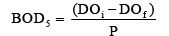(1.6.3)
Where, DOi and DOf are initial and final DO concentrations of the diluted sample, respectively. P is called as dilution factor and it is the ratio of sample volume (volume of wastewater) to total volume (wastewater plus dilution water). In the above formula, it was assumed that the diluted wastewater had no oxygen demand of itself and that the dilution wastewater used was pure.

Most of the times, microorganisms are added in the dilution water (seeded water) so as to have enough microorganisms for carrying out biodegradation of organic waste. In this case, the oxygen demand of seeded water is subtracted from the demand of mixed sample of waste and dilution water. In this case,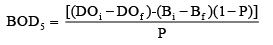(1.6.4)
Where, Bi and Bf are initial and final DO concentrations of the seeded diluted water (blank).

Modeling BOD as first order reaction

Assuming that the rate of decomposition of organic waste is proportional to the waste left in the flask: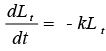(1.6.5)
Where, Lis the amount of oxygen demand left after time t and k is the BOD rate constant (time-1). Solving this equation yields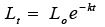(1.6.6)
Where, Lo is the ultimate carbonaceous oxygen demand and it is also the amount of O2 demand left initially (at time 0, no DO demand has been exerted, so BOD = 0)  At any time, Lo = BODt + Lt (that is the amount of DO demand used up and the amount of DO that could be used up eventually). Assuming that DO depletion is first order: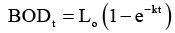(1.6.7)

As temperature increases, metabolism increases, utilization of DO also increases, therefore, k is a function of temperature (T in oC). k at any temperature T (oC) is obtained as: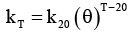(1.6.8)
Where, k 20 is the value of k at 20oC and θ is an empirical constant. θ = 1.135 if T is between 4 - 20oC; θ = 1.056 if T is between 20 - 30oC.

CHEMICAL OXYGEN DEMAND (COD)

This test is carried out on the sewage to determine the extent of readily oxidizable organic matter, which is of two types:
a. Organic matter which can be biologically oxidized is called biologically active
b. Organic matter which cannot be oxidized biologically is called biologically inactive. COD gives the oxygen required for the complete oxidation of both biodegradable and non-biodegradable matter.

• COD is a measure of the oxygen equivalent of the organic matter content of a sample that is susceptible to oxidation by a strong chemical oxidant.
• It is an indirect method to measure the amount of organic compounds in water.
• It is expressed in milligrams per liter (mg/L), which indicates the mass of oxygen consumed per liter of solution.

Analytical Procedure  Organic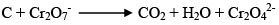(1.6.9)

• A sample is refluxed in strongly acidic solution with a known excess of potassium dichromate (K2Cr2O7) for 2-3 h.
• After digestion, the remaining unreduced K2Cr2O7 is titrated with ferrous ammonium sulphate to determine the amount of K2Cr2O7 consumed.
• Then, the oxidizable matter is calculated in terms of oxygen equivalent.
• This procedure is applicable to COD values between 40 and 400 mg/L.

Essential differences between BOD and COD

• COD always oxidize things that the BOD cannot or will not measure; therefore, COD is always higher than the BOD. The common compounds which cause COD to be higher than BOD include sulfides, sulfites, thiosulfates and chlorides.
• The general relationship between BOD and COD for sewage and most human wastes is about 1 unit of BOD≈0.64–0.68 units of COD. The relationship is not consistent and it may vary considerably for industrial wastewaters.
The document Water Quality Monitoring: Estimation of Alkalinity, BOD & COD Notes | Study Environmental Engineering - Civil Engineering (CE) is a part of the Civil Engineering (CE) Course Environmental Engineering.
All you need of Civil Engineering (CE) at this link: Civil Engineering (CE)Use Code STAYHOME200 and get INR 200 additional OFF

## Environmental Engineering

14 videos|74 docs|70 tests

Track your progress, build streaks, highlight & save important lessons and more!

,

,

,

,

,

,

,

,

,

,

,

,

,

,

,

,

,

,

,

,

,

,

,

,

;